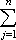#Interactive Real Analysis

Next | Previous | Glossary | Map

## 7.1. Riemann Integral

### Examples 7.1.15:

Find a function that is not integrable, a function that is integrable but not continuous, and a function that is continuous but not differentiable.

What this example really shows is

• there are functions that are not integrable, continuous, or differentiable
• there are more Riemann integrable functions than there are continuous functions
• there are more continuous functions than there are differentiable ones

A function that is not integrable:

Take the Dirichlet function. We have previously shown that I*(f) = 1 and I*(f) =0. Therefore the Dirichlet function is not integrable.

A function that is integrable but not continuous:

Take the function that equals 1 over the interval [0, 1] and 2 over the interval (1, 2]. It is clear that the function is not continuous, but we need to prove that it is integrable.

Take a partition P of the interval [0, 2] with norm less than. If the point 1 = xk is part of the partition we have:

| U(f, P) - L(f, P)||cj - dj| (xj - xj-1)
= |ck - dk| (xk - xk-1) + |ck+1 - dk+1| (xk+1 - xk) =
= | 1 - 1 | (1 - xk-1) + |2 - 1| (xk+1 - 1) =
= xk+1 - 1 =
= xk+1 - xk <because cj = dj over all subintervals except one that includes xk. If, on the other hand, the point 1(xk-1, xk) is not part of the partition we have:
| U(f, P) - L(f, P)||cj - dj| (xj - xj-1)
= |ck - dk| (xk - xk-1) =
= | 2 - 1 | (xk - xk-1) =
= xk - xk-1 <because again cj = dj over all subintervals except the one that includes the point 1. Either way, Riemann's Lemma now says that f is integrable.
A function that is continuous but not differentiable:
Take the absolute value function f(x) = |x| over the interval [-1, 1]. It is integrable because it is continuous, but not differentiable because it has a sharp corner at 0.
Next | Previous | Glossary | Map